Contents >> Applied Mathematics >> Numerical Methods >> Ordinary Differential Equations >> Runge-Kutta method

 Ordinary differential equations - Runge-Kutta method Runge- Kutta method Let’s again consider the differential equation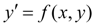(1) with an initial condition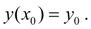The classical 4-th order Runge-Kutta method is described by the following system of five equalities: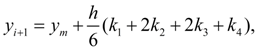(5) where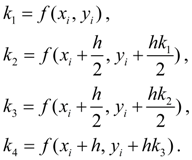Strictly speaking, there is not one, and group of Runge-Kutta methods differing from each to other by order, i.e. quantity of parameters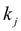. In this case we have the 4-th order method, which is one of the most put into practice as provides high accuracy and at the same time differs comparative simplicity. Therefore in most cases it is mentioned in the literature simply as «Runge-Kutta method» without the instruction of its order.

 < Previous Contents Next >